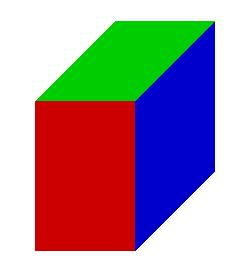# ABCDA'B'C'D 6261

The ABCDA'B'C'D 'prism has a square base. The wall diagonal of the AC base is 9.9 cm long, and the body diagonal AC 'is 11.4 cm long. Calculate the surface area and volume of the prism.

S =  256.2862 cm2
V =  276.9975 cm3

### Step-by-step explanation:Did you find an error or inaccuracy? Feel free to write us. Thank you!

Tips for related online calculators
Are you looking for help with calculating roots of a quadratic equation?
Do you have a linear equation or system of equations and looking for its solution? Or do you have a quadratic equation?
The Pythagorean theorem is the base for the right triangle calculator.